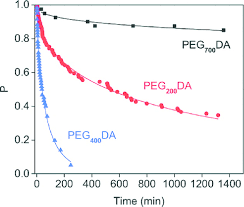## How to Calculate and Solve for P(A or B) | ProbabilityThe image above represents P(A or B).

To compute for P(A or B), four essential parameters are needed and these parameters are xA, NA, xB and NB.

The formula for calculating P(A or B):

P(A or B) = P(A) + P(B)

Where;

P(A) =  xANA
P(B) = xBNB

Let’s solve an example;
Find the P(A or B) when xA is 9, NA is 11, xB is 6 and NB is 12.

This implies that;

xA = 9
NA = 11
xB = 6
NB = 12

P(A or B) = P(A) + P(B)
P(A or B) = xANA + xBNB
P(A or B) = 911 + 612
P(A or B) = 9(12) + 6(11)(11)(12)
P(A or B) = 108 + 66132
P(A or B) = 174132
Dividing the numerator and denominator by 6
P(A or B) = 2922
P(A or B) = 1.318

Therefore, the P(A or B) is 1.318.